# Transistor Characteristics

In physics, the graph representing the relationships between the current and the voltage of any transistor of any configuration is called Transistor Characteristics. In this session, let us learn the transistor characteristics in detail.

## Characteristics of Transistor

Any two-port network which is analogous to transistor configuration circuits can be analyzed using three types of characteristic curves. They are

• Input Characteristics: The curve describes the changes in the values of input current with respect to the values of input voltage, keeping the output voltage constant.
• Output Characteristics: The curve is obtained by plotting the output current against output voltage, keeping the input current constant.
• Current Transfer Characteristics: This characteristic curve describes the variation of output current in accordance with the input current, keeping the output voltage constant.

## Configuration of Transistor

Any transistor circuit can be designed using three types of configuration. Three configurations of the transistor are based on the connection of the transistor terminal. The three types of transistor circuit configurations are:

• Common Emitter Transistor
• Common Base Transistor
• Common Collector Transistor(emitter follower).

Each of these three circuit configurations has its own characteristics curve. Based on the requirement the type will be chosen for the circuit.

You may also want to check out these topics given below!

### Common Emitter (CE) Configuration of Transistor

The configuration in which the emitter is connected between the collector and base is known as a common emitter configuration.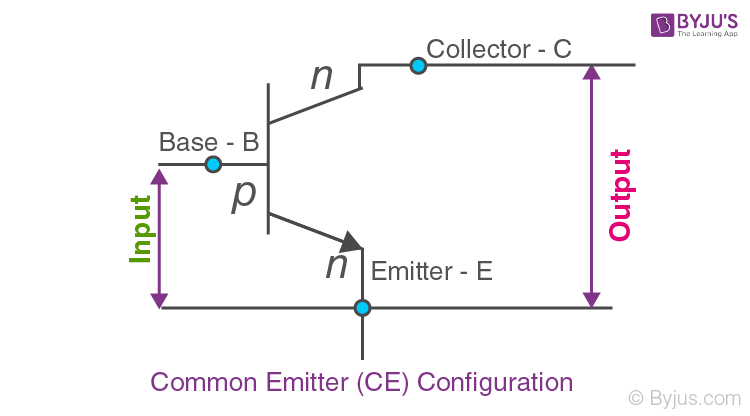Common Emitter (CE) Configuration of Transistor

The transistor characteristic under Common Emitter configuration is as follows:

 Transistor Characteristics Definition Formula/Expression Characteristic Curve Input Characteristics The variation of emitter current(IB) with Base-Emitter voltage(VBE), keeping Collector Emitter voltage(VCE) constant. $$\begin{array}{l}R_{in}=\frac{\Delta V_{BE}}{\Delta I_{B}}|V_{CE}=Constant\end{array}$$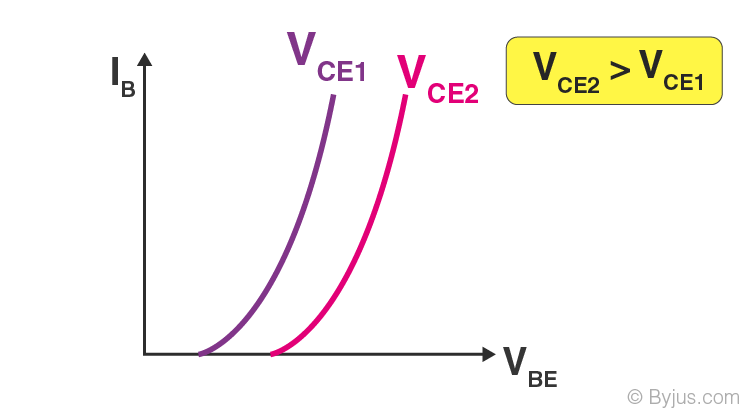Output Characteristics The variation of collector current(IC) with Collector-Emitter voltage(VCE), keeping the base current(IB) constant. $$\begin{array}{l}R_{out}=\frac{\Delta V_{CE}}{\Delta I_{C}}|I_{B}=Constant\end{array}$$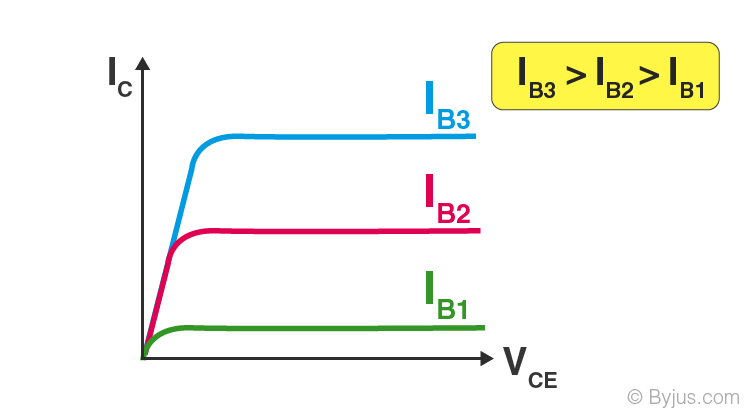Current Transfer Characteristics The variation of collector current(IC) with the base current(IB), keeping Collector-Emitter voltage(VCE) constant. The resulting current gain has a value greater than 1. $$\begin{array}{l}\alpha =\frac{\Delta I_{C}}{\Delta I_{B}}|V_{CB}=Constant\end{array}$$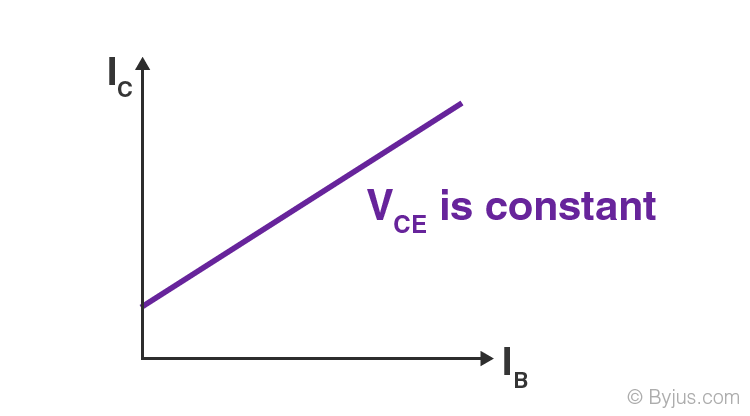### Common Base (CB) Configuration of Transistor

In CB Configuration, the base terminal of the transistor will be connected common between the output and the input terminals.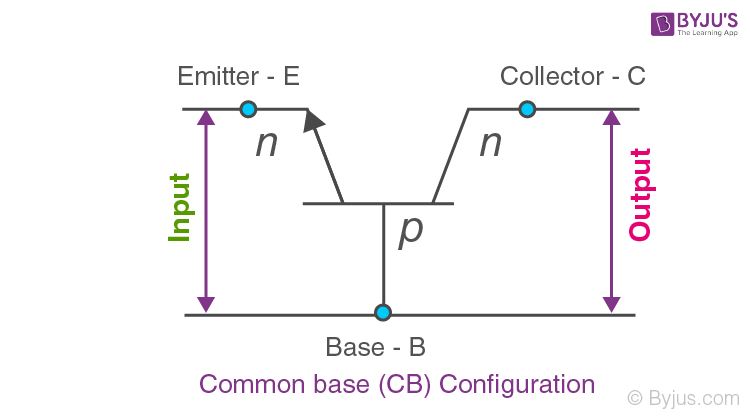The transistor characteristic under Common Base configuration is as follows:

 Transistor Characteristics Definition Formula/Expression Characteristic Curve Input Characteristics The variation of emitter current(IE) with Base-Emitter voltage(VBE), keeping Collector Base voltage(VCB) constant. $$\begin{array}{l}R_{IN}=\frac{\Delta V_{BE}}{\Delta I_{E}}|V_{CB}=Constant\end{array}$$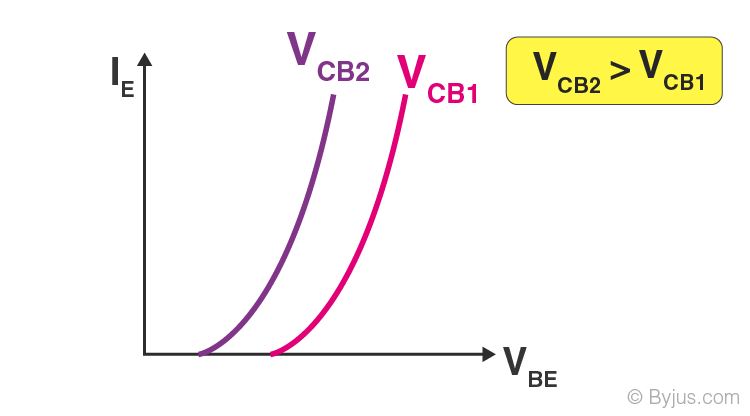Output Characteristics The variation of collector current(IC) with Collector-Base voltage(VCB), keeping the emitter current(IE) constant. $$\begin{array}{l}R_{OUT}=\frac{\Delta V_{CB}}{\Delta I_{B}}|I_{E}=Constant\end{array}$$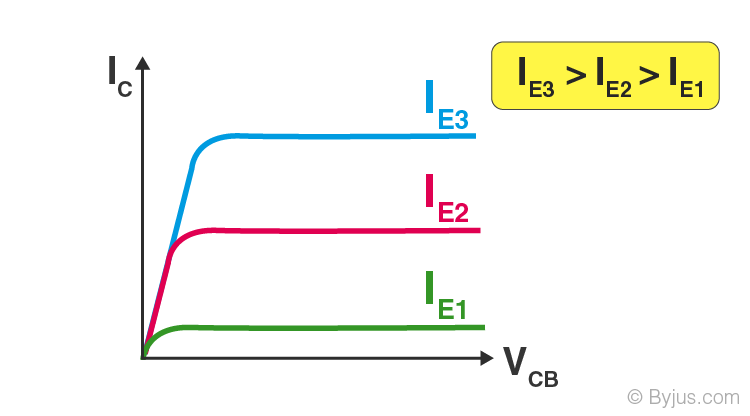Current Transfer Characteristics The variation of collector current(IC) with the emitter current(IE), keeping Collector Base voltage(VCB) constant. The resulting current gain has a value less than 1. $$\begin{array}{l}\alpha =\frac{\Delta I_{C}}{\Delta I_{B}}|V_{CB}=Constant\end{array}$$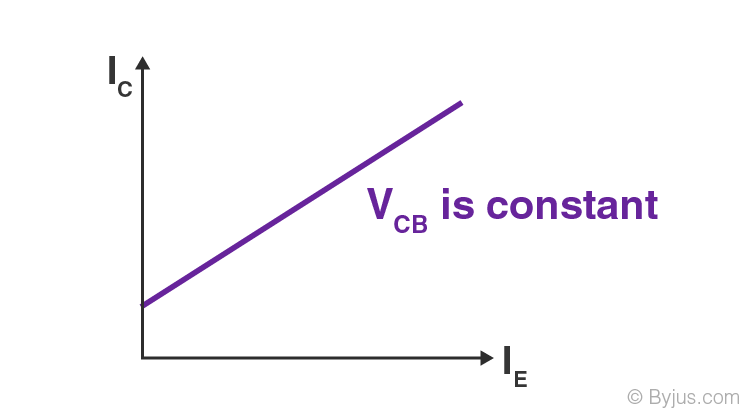### Common Collector (CC) Configuration of Transistor

In CE Configuration, the Collector terminal of the transistor will be connected common between the output and the input terminals.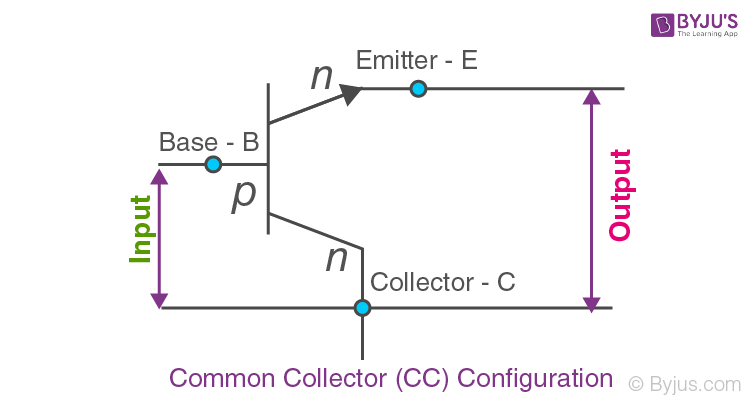The transistor characteristic under Common Collector configuration is as follows:

 Transistor Characteristics Definition Characteristic Curve Input Characteristics The variation of emitter current(IB) with Collector-Base voltage(VCB), keeping Collector Base voltage(VCB) constant.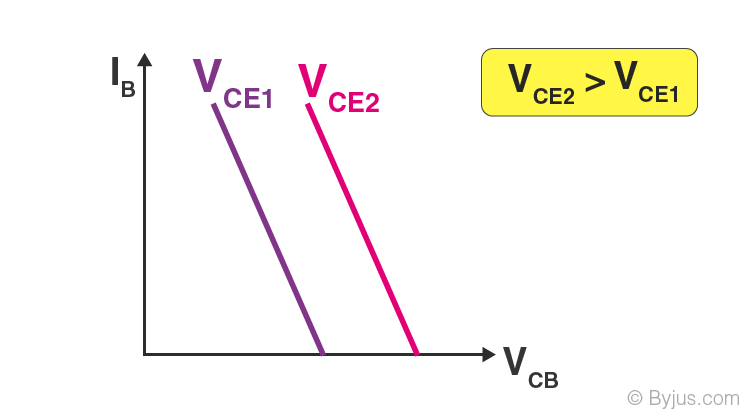Output Characteristics The variation of emitter current(IE) with Collector-Emitter voltage(VCE), keeping the base current(IB) constant.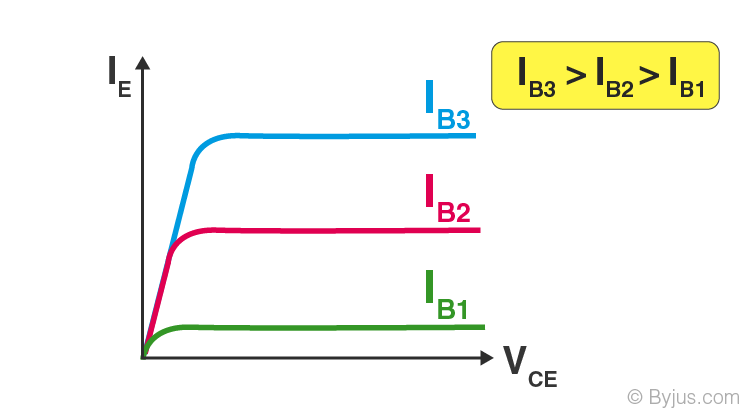Current Transfer Characteristics The variation of Emitter current(IE) with the base current(IB), keeping Collector-Emitter voltage(VCE) constant.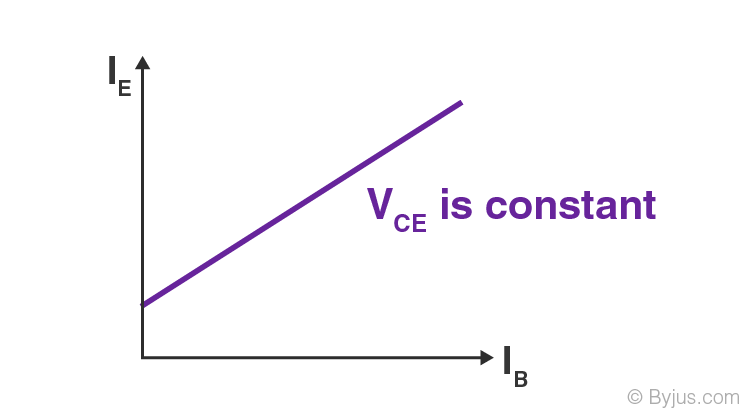## Frequently Asked Questions – FAQs

Q1

### What is a transistor?

A transistor is a semiconductor device used to conduct and insulate electric current or voltage.

Q2

### What are the three terminals of a transistor?

There are three terminals for a transistor are:

• Base
• Collector
• Emitter
Q3

### What are the types of transistor circuit configurations?

Types of transistor circuit configurations are:
• Common Emitter Transistor
• Common Base Transistor
• Common Collector Transistor
Q4

### What is a PNP Transistor?

PNP Transistor is a type of transistor where one n-type material is placed between two p-type materials.
Q5

### Define the current transfer characteristics?

The current transfer characteristics curve defines the variation of output current in accordance with the input current, keeping the output voltage constant.

Stay tuned with BYJU’S for more such interesting articles. Also, register to “BYJU’S-The Learning App” for loads of interactive, engaging physics-related videos and unlimited academic assistance.

Test your knowledge on Characteristics of transistor